Courses

# Test: Square Root And Cube Root- 1

## 10 Questions MCQ Test Logical Reasoning (LR) and Data Interpretation (DI) | Test: Square Root And Cube Root- 1

Description
This mock test of Test: Square Root And Cube Root- 1 for Quant helps you for every Quant entrance exam. This contains 10 Multiple Choice Questions for Quant Test: Square Root And Cube Root- 1 (mcq) to study with solutions a complete question bank. The solved questions answers in this Test: Square Root And Cube Root- 1 quiz give you a good mix of easy questions and tough questions. Quant students definitely take this Test: Square Root And Cube Root- 1 exercise for a better result in the exam. You can find other Test: Square Root And Cube Root- 1 extra questions, long questions & short questions for Quant on EduRev as well by searching above.
QUESTION: 1

### Q.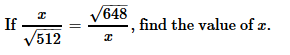Solution: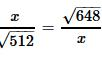⇒ x=  √331776

⇒ x2 = 576

⇒ x = √576

⇒ x = 24

QUESTION: 2

###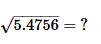Solution:

(5.4756)1/2 = 2.34

QUESTION: 3

### If 3√5 + √125 = 17.88, then what will be the value of √80 + 6√5?

Solution: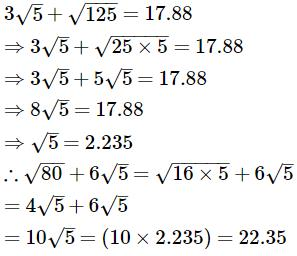QUESTION: 4

The cube root of 0.000729 is

Solution: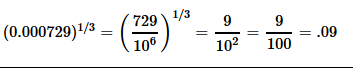QUESTION: 5

What is the least perfect square which is divisible by each of 21, 36 and 66?

Solution:

L.C.M. of 21, 36, 66 = 2772

Now, 2772 = 2 x 2 x 3 x 3 x 7 x 11

To make it a perfect square, it must be multiplied by 7 x 11.

So, required number = 2 x 2 x 3 x 3 x 7 x 7 x 11 x 11 = 213444

QUESTION: 6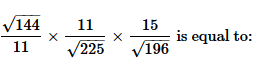Solution: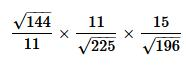= 12/11 x 11/15 x 15/14

= 12/14

= 0.85

QUESTION: 7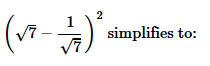Solution: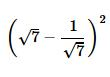Using identity, (a-b)2 = a2 + b2 - 2ab

Here a = √7 and b = 1/√7= (√7)+ (1/√7)2 - 2. √7.1/√7

= 7 + 1/7 - 2

= 5 + 1/7

= 36/7

QUESTION: 8

The square root of 16641 is

Solution:

Step 1 : We now need to obtain the digital root of the number. Here's how you do it:
Split the number up and add each digit together:
1 + 6 + 6 + 4 + 1 = 18
If the answer is more than one digit, you would add each digit of the answer together again:
1 + 8 = 9
What is the digital root of number 16,641?
Step 2:
So now we know the digital root of 16,641 is 9. Is 9 in the list of digital roots that are always a square root (1, 4, 7 or 9)?
Answer: YES, 9 is in the list of digital roots that are always perfect squares. We can conclude that 16,641 could be a perfect square!
Factoring
OK, so now we know that 16,641 could be a perfect square. We have to find the factors of the number to be sure.
Here are all of the factors of 16,641:
(1 x 16,641) (3 x 5,547) (9 x 1,849) (43 x 387) (129 x 129)
Hence the answer is 129 x 129

QUESTION: 9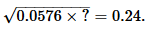Solution: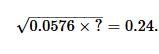Squaring both side,

⇒ 0.0576 x a = 0.0576

⇒ a = 1

QUESTION: 10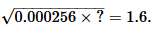Solution: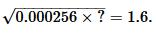Squaring both side,

⇒ 0.000256 x a = 2.56

⇒ a = 2.56/0.000256

⇒ a = 10000